• matlab频率域图像处理代码和实验报告-实验、频率域图像处理.rar 频率域图像处理 实验三、频率域图像处理 （一）实验目的： 1、理解图像的傅立叶变换； 2、掌握频率域滤波的基本方法。 （二）实验要求： 1...matlab
• 基于MATLAB图像处理的频率域滤波分析及应用.pdf
• 基于频率域平滑滤波的数字图像处理方法研究论文，附源代码，能够实现图像的频率域平滑滤波效果，适用于图像去噪等。
• 通过MATLAB进行频率域图像增强实验，注意文件和图片在同一目录下
• 图像增强处理：设计一套空间域与频率域结合的图像增强算法，处理以下任一组图片中的带噪声图像，去除噪声，提高图像质量。 （1）已知：噪声为随机噪声和周期噪声混合噪声； （2）要求： a）去噪处理后，计算均方误差...
• 本文利用能量损失的概念，对二度体异常取有限长剖面及离散数据作向上延拓、向下延拓及化到磁极，特别是向上延拓后对异常的影响作了研究，提出了确定剖面长度及采样点距的一般公式。
• dft2d函数为对灰度图进行离散傅里叶变换和反变换 filter2d_freq为对灰度图进行频率域滤波 修改Runner函数中的图片路径然后运行即可
• 在不使用matlab的情况下，可以选择用python来实现自动控制理论有关系统打时域分析和频率域分析等，安装的package是python-control，在windows的控制台(cmd)或者linux终端下输入pip install control 即可，注意，如果...
• 分析二维数据在频率-空间频率-波数、拉东的特征，适用于地震、雷达、超声数据的分析
• 内容简介频率域去噪带阻滤波器带通滤波器陷波滤波器最佳陷波滤波器 频率域去噪 为了节约读者时间，首先说明这一篇不会有任何代码只有个人的心得体会理由如下： 代码过于简单，像书上这样的例子这样的 自己并没有这类...
• 首先，把多幅具有互补信息的低分辨率图像通过傅里叶变换到频率域；接着，对频率域中高分辨与低分辨率图像之间关系进行建模，进而得到它们之间的关系；然后，通过对模型求解，得到高分辨率图像的频率值；最后，进行...傅里叶变换 超分辨恢复 亚像素
• 计算机编号 电子信息学院 实验报告书 课 程 名 数字图像处理课程实验 题 目实验四 空间域和频率域图像处理 实验类别 验证实验 班 级 电子 1313 学 号 131003430326 姓 名 吴限 评语 1实验程序代码 正确 基本正确 不...
• matlab开发-频率域自适应滤波器。执行自正交频域自适应滤波器一次迭代的函数。
• 本文档包含数字图像处理(冈萨雷斯第三版)中的图像频率域滤波（傅里叶变换、巴特沃斯低通滤波高通滤波等）实验，包含整个实验过程和原理解释，以及详细的执行代码。代码复制后可在matlab中直接运行。
• 频率域的互相关计算速度比时间域快很多，是一种非常好的实现互相关计算的算法。
• 对于实际生产中遇到的2.5维问题,从麦克斯韦方程组出发,把地电参数变化小的走向方向转化到波数,用一系列波数模拟三维源的特征,并在波数中,得到2组关于Hx(kx,y,z)和Ex(kx,y,z)的偏微分方程。选取适当的kx值,用有限...
• ## 频率域滤波

千次阅读 2019-11-11 19:38:34
之前介绍了关于空间域滤波的一些操作，主要目的是减少噪声和平滑图像，空间域的处理方法都是在已知噪声数据类型基础上进行噪声过滤的，而在某些噪声图像类别上上述方法就显得十分渺小了，频率域的引入就是来寻找噪声...
之前介绍了关于空间域滤波的一些操作，主要目的是减少噪声和平滑图像，空间域的处理方法都是在已知噪声数据类型基础上进行噪声过滤的，而在某些噪声图像类别上上述方法就显得十分渺小了，频率域的引入就是来寻找噪声的类型，并进行空间过滤的。其中频率是单位时间变化期间，一个周期函数重复相同值序列的次数。频率域滤波(即波数)为图像像元的灰度值随着位置变化的空间频率，以频谱表示信息分布特征，可以将一幅图像像元值在空间上的变化分解为 具有不同振幅、空间频率和相位的简振函数的线性叠加，图像中各种空间频率成分的组成和分布称为空间频谱。这种对图像的空间频率特征进行分解、处理和分析称为频率域处理。频率中最典型的就是傅立叶变换，傅立叶变换能把图像从空间域变换到只包含不同频率信息的频率域，原图像上的灰度突变部位、图像结构复杂的区域、图像细节及干扰噪声等信息集中在高频区，而原图像上灰度变化平缓部位的信息集中在低频区(变换函数还有小波变换，将在后续进行分析和解析)。
傅立叶变换
傅立叶变换是将时间域转换为频率域的工具，傅立叶指出任何周期或函数都可以表示为不同频率的正弦和/或余弦之和的形式，每个正弦和/或余弦乘以不同的系数（称该和为傅立叶级数）。无论函数多么复杂，只要它是周期的，并且满足一定条件，都可以用这样的和表示。一维变换函数（一对，变换及反变化函数）为：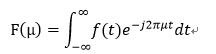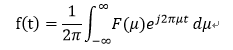其中，变换后的的函数F(x)，f(t)为变换前周期函数，μ为周期函数分量，周期从0到1/2π。
利用一维变量扩展到二维变量，二维傅立叶变换公式为：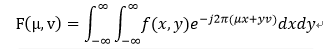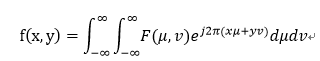e^j2π(xμ+yv)实际上是一个平面波（的共轭），它的传播方向与(u,v)相同，角频率是 根号下x^2+y^2。可以看做是平面波在x方向的角频率是u和y方向的角频率是v。二维傅立叶变换具有旋转不变性了（证明：如何证明二维离散傅里叶变换的旋转不变性？）。同时，变换完，中心是两个角频率都为0（直流分量），越靠近中心，两个方向的角频率越低，合成的角频率与到中心的距离成正比，所以中心是低频分量，外部是高频分量。幅度决定图像的强弱，相位觉得图像的像素。分析
频率域滤波基本步骤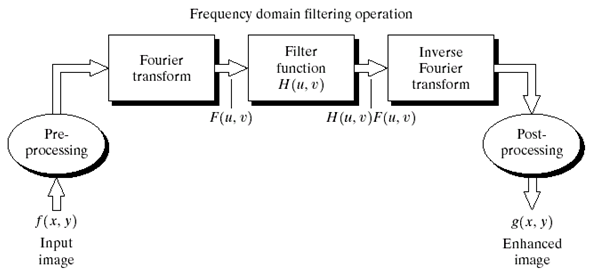preprocessing：图像预处理步骤，用0填充f(x,y)，在二维循环卷积时为了保证周期性进行0填充（傅里叶变换滤波时，为什么需要对输入数据进行零填充？）
Fourier transform：图像傅立叶变换
filter function：滤波器
Inverse Fourier transform：反向傅立叶变换
postprocessing：图像裁剪，输出g(x,y)
伪流程：
1.f(x,y)是由MxN构成，填充图像大小为P(P=2M)xQ(Q=2N)，f_p(x,y)
2.将f_p(x,y)利用-1^(x+y)将图片滤波器移动到中心，低频部分移到中间，高频部分移到四周，以便后面的计算。
3.计算f_p(x,y)傅立叶变化得到F(u,v)
4.生成一个实的、对阵的过滤器，中心在（P/2,Q/2），大小为PxQ
5.计算H(u,v)F(u,v)，并进行换位，得到初始时图像的傅立叶变换（DEF）的数据排列形式，即低频部分在四周，高频部分在中间（原点在左上角意味着低频部分在左上角，又因为的DEF是中心对称的，可得初始时图像的DEF应是低频在四周高频在中间）。
6.计算反傅立叶函数，得到每个元素的幅度（实部和虚部平方和的平方根），并取左上角MxN区域，得到最终的输出图像g(x,y)。
过滤器
将一幅图像的模糊化（频率域平滑）可通过高频的衰减来达到，称为低通滤波器。
将一幅图像的锐利化（边缘聚变与高频有关）可通过低频的衰减来达到，称为高通滤波器。
a.低通滤波器
（1）理想低通滤波器
在以原点为圆心，以为半径的园内，无衰减地通过所有频率，而在该圆外"切断"所有频率的二维低通滤波器，称为理想低通滤波器，它由下面函数确定：

其中，D(u,v)是频率域中点(u,v)与频率矩阵中心的距离，即：

图4.40(a)中是H(u,v)的透视图，图b显示了以图像显示的滤波器，表明在半径D0的圆内，所有频率都无衰减的通过，而在此圆外的所有频率则是完全被衰减（滤除）。理想低通滤波器是关于原点径向对称的，这意味着该滤波器完全是一个径向横截面来定义，将图绕着H(u,v) 360度旋转则得到图a。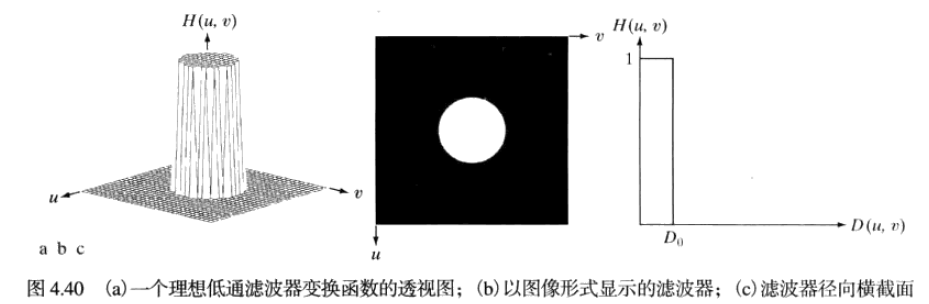（2）布特沃斯低通滤波器
截止频率位于距原点D0处的n阶布特沃斯低通滤波器（BLPF）的传递函数定义如下：
BLPF传递函数并没有在通过频率和滤除频率之间给出明显截止的尖锐的不连续性。图4.44(a)是透视图，表明在半径D0的圆内，频率是有规则的通过，而在此圆外的所有频率则是完全被衰减（滤除）。在空间域的一阶布特沃斯滤波器没有振铃现象，在二阶滤波器中，振铃现象通过很难察觉（通过设置不同的n值，二阶的BLPF是在有效的低通滤波和可接受的振铃特性之间好的折中），单更高阶数的滤波器中振铃现象会很明显。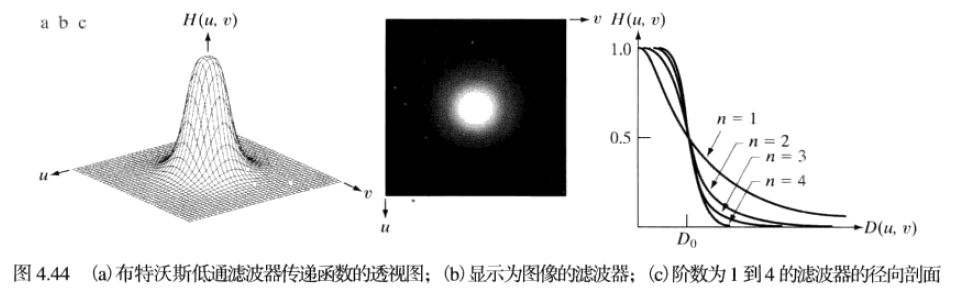（3）高斯低通滤波器
二维高斯低通滤波器（GLPF）形式如下：

其中D(u,v)是距离频率矩形中心的距离，是关于中心扩展度的度量。图4.47显示了一个GLPF函数的透视图、图像显示和径向剖面图。GLPF的傅立叶反变换也是高斯的，从图a看得到的空间高斯滤波器没有振铃。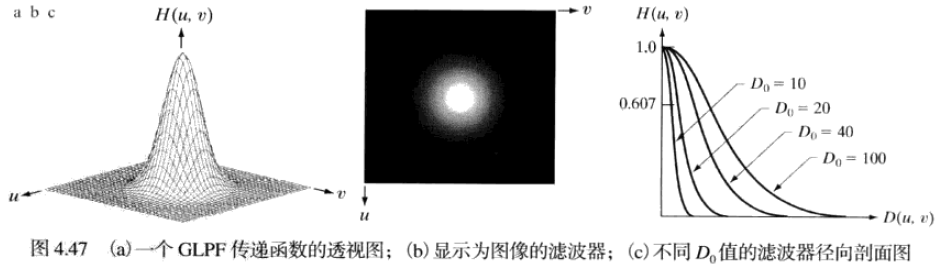b.高通滤波器
通过衰减图像的傅立叶变换的高频成分可以平滑图像。因为边缘和其他灰度的急剧变化与高频分量有关，所以图像的锐化可在频率域通过高通滤波来实现，高通滤波会衰减傅立叶变换中的低频分量而不会扰乱高频信息。
（1）理想高通滤波器
一个二维理想高通滤波器（IHPF）定义为

其中是截止频率，D(u,v)是频率域中点(u,v)与频率矩阵中心的距离。在这种滤波器下，IHPF把以半径为的圆内的所有频率置0，而毫无衰减地通过圆外的所有频率。和理想低通过滤器完全相反。下图为理想高通滤波器的透视图、图像表示和剖面图。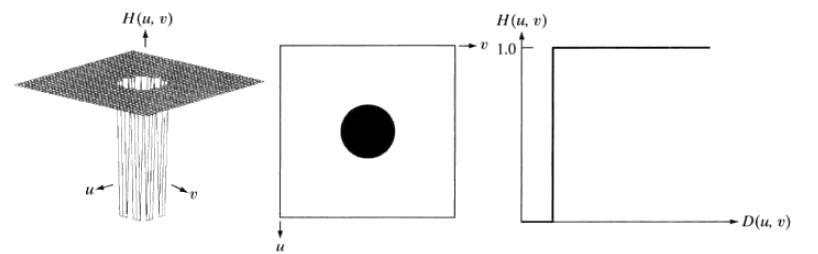（2）布特沃斯高通滤波器
截止频率的n阶布特沃斯高通滤波器(BHPF)定义为：

类似于低通滤波器，BHPF比IHPF更平滑一些。下图为布特沃斯高通滤波器的透视图、图像表示和剖面图。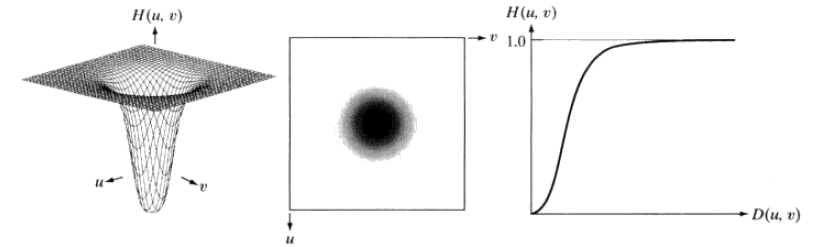（3）高斯高通滤波器
截止频率出在距频率矩形中心距离为的高斯高通滤波器(GHPF)的传递函数：

类似高斯低通滤波器，高斯高通滤波器比理想高通滤波器、布特沃斯高通滤波器平滑效果更好一些。即使是对微小物体和细线条使用高斯滤波器滤波，结果也是比较清晰的。下图为高斯高通滤波器的透视图、图像表示和剖面图。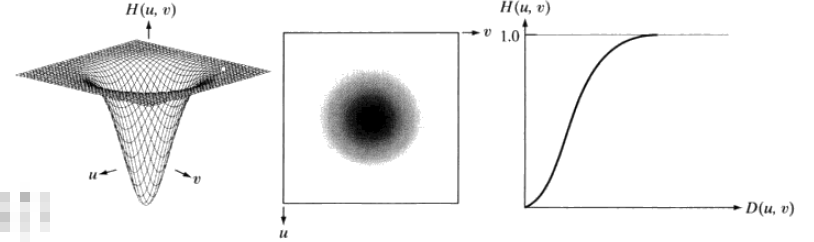https://www.cnblogs.com/laumians-notes/p/8544256.html
https://blog.csdn.net/liuweiyuxiang/article/details/77040942
https://blog.csdn.net/nanhuaibeian/article/details/90738701
https://blog.csdn.net/chanxiaogang/article/details/45226373
https://blog.csdn.net/eeeee123456/article/details/82950986
展开全文滤波器 傅立叶变换
• 该程序为利用matlab进行图像处理的代码，主要针对傅里叶正反变换以及其频率域的呈现，以及理想（方形）低通滤波器、Butterworth滤波器的实现，代码费了不少心思，毕竟八百年没碰过matlab了，竟然写到一半开始拼...
• 为此，在分析了黑色背景图像上叠加规则白色形状的同态傅里叶变换的基础上，提出了一种基于频率域的图像质量评价模型。该模型通过对频率域所表现出的图像纹理结构进行预处理，最终建模实现了高通量基因测序图像质量的...
• 该程序是CG杂志作者上传的代码，主要用于GEM2频率域电磁数据的反演成像，通过修改也可以用于航空电磁，供参考
• 【项目程序】对图像进行频率域图像增强，计算并画出此图像的中心化频率谱，用拉普拉斯算子对此图像锐化.rar图像
• 一、原理_频率域锐化 理想高通滤波器的传递函数为： n 阶巴特沃斯高通滤波器的传递函数为： n 阶指数高通滤波器的传递函数为： 二、步骤 (1)读入原图像test.tif并显示； (2)采用理想高通滤波器对图像滤波，并显示...
一、原理_频率域锐化
理想高通滤波器的传递函数为：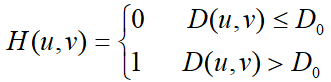n 阶巴特沃斯高通滤波器的传递函数为：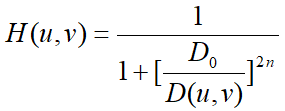n 阶指数高通滤波器的传递函数为：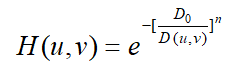二、步骤
(1)读入原图像test.tif并显示； (2)采用理想高通滤波器对图像滤波，并显示滤波结果； 观察截止频率 D0 分别为15、30、80时，理想高通滤波的结果。 (3)采用二阶巴特沃斯高通滤波器对图像滤波，并显示滤波结果； 观察截止频率 D0 分别为15、30、80时，二阶巴特沃斯高通滤波的结果。 (4)采用二阶指数高通滤波器对图像滤波，并显示滤波结果； 观察截止频率 D0 分别为15、30、80时，二阶指数高通滤波的结果。 (5)比较各种高通滤波器的滤波效果。
三、实验图像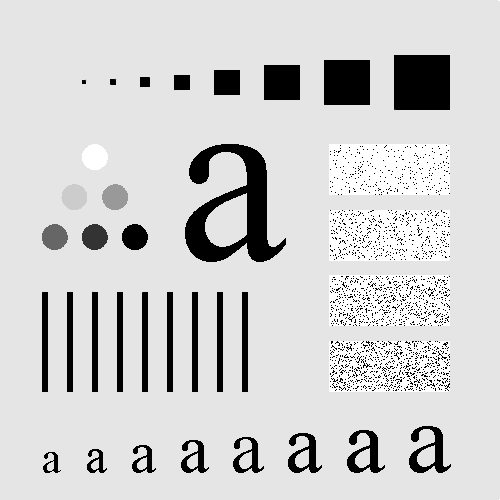test.tif
将图片存到路径：D:\test.tif
四、框图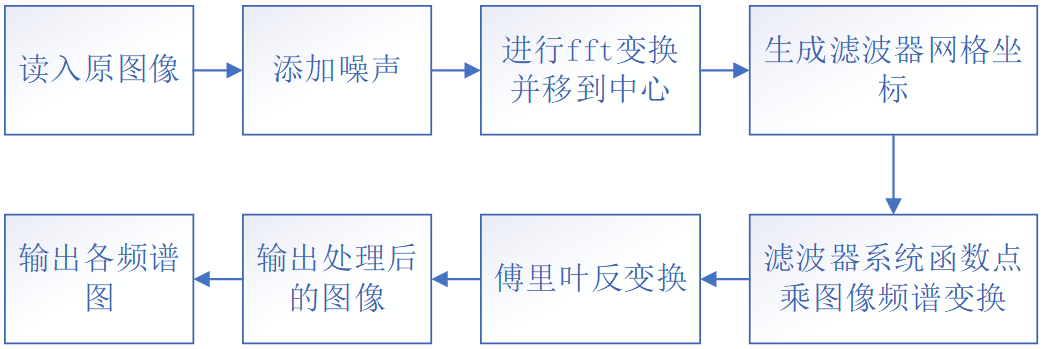五、代码
%------------------------------------------------------------------------
% File name:           second_3
% Author:              Jasmine
% Descriptions:        频率域锐化
%------------------------------------------------------------------------

%清空工作区
clc,clear,close all;
%获取图像
img_noise = imnoise(img, 'gaussian', 0, 0.02);
I = im2double(img_noise);
[m, n] = size(I);
n2 = 2*n;
m2 = 2*m;
%%%%%%%%%%%%%%%%%%%%%%%%%%%%%%%%%%%%%%%%%%%%%%%%%%%%%%%%%%%%%%%%%%%%%%%%%%%
%进行fft变换并移到中心
F = fftshift(fft2(I, m2, n2));
figure(1);
subplot(4,3,2),imshow(mat2gray(log(1+abs(F)))),title('加噪图像 频谱图')
%%%%%%%%%%%%%%%%%%%%%%%%%%%%%%%%%%%%%%%%%%%%%%%%%%%%%%%%%%%%%%%%%%%%%%%%%%%
%设计滤波器
%生成网格坐标
u = -n:n-1;
v = -m:m-1;
[U,V] = meshgrid(u,v);
D = hypot(U, V);
D0 = 15;D1 = 30;D2 = 80;   %[15, 30, 80]
%各滤波器的系统函数
H11 = mat2gray( D >= D0 );%理想高通滤波器截止频率为15
H12 = mat2gray( D >= D1 );%理想高通滤波器截止频率为30
H13 = mat2gray( D >= D2 );%理想高通滤波器截止频率为80
H21 = mat2gray(1./(1+((D0./D).^4)));%巴特沃斯高通滤波器截止频率为15
H22 = mat2gray(1./(1+((D1./D).^4)));%巴特沃斯高通滤波器截止频率为30
H23 = mat2gray(1./(1+((D2./D).^4)));%巴特沃斯高通滤波器截止频率为80
H31 = mat2gray(exp(-(D0./D).^2));%指数高通滤波器截止频率为15
H32 = mat2gray(exp(-(D1./D).^2));%指数高通滤波器截止频率为30
H33 = mat2gray(exp(-(D2./D).^2));%指数高通滤波器截止频率为80
%滤波器的系统函数与图像频谱变换相乘
G11 = F.*H11;
subplot(4,3,4);imshow(mat2gray(log(1+abs(G11))));title('理想高通滤波15 频谱图');
G12 = F.*H12;
subplot(4,3,5);imshow(mat2gray(log(1+abs(G12))));title('理想高通滤波30 频谱图');
G13 = F.*H13;
subplot(4,3,6);imshow(mat2gray(log(1+abs(G13))));title('理想高通滤波80 频谱图');
G21 = F.*H21;
subplot(4,3,7);imshow(mat2gray(log(1+abs(G21))));title('巴特沃斯高通滤波15 频谱图');
G22 = F.*H22;
subplot(4,3,8);imshow(mat2gray(log(1+abs(G22))));title('巴特沃斯高通滤波30 频谱图');
G23 = F.*H23;
subplot(4,3,9);imshow(mat2gray(log(1+abs(G23))));title('巴特沃斯高通滤波80 频谱图');
G31 = F.*H31;
subplot(4,3,10);imshow(mat2gray(log(1+abs(G31))));title('指数高通滤波15 频谱图');
G32 = F.*H32;
subplot(4,3,11);imshow(mat2gray(log(1+abs(G32))));title('指数高通滤波30 频谱图');
G33 = F.*H33;
subplot(4,3,12);imshow(mat2gray(log(1+abs(G33))));title('指数高通滤波80 频谱图');
%傅里叶反变换
g011 = ifft2(fftshift(G11));g11 = g011(1:m,1:n);g11 = real(g11);
g012 = ifft2(fftshift(G12));g12 = g012(1:m,1:n);g12 = real(g12);
g013 = ifft2(fftshift(G13));g13 = g013(1:m,1:n);g13 = real(g13);
g021 = ifft2(fftshift(G21));g21 = g021(1:m,1:n);g21 = real(g21);
g022 = ifft2(fftshift(G22));g22 = g022(1:m,1:n);g22 = real(g22);
g023 = ifft2(fftshift(G23));g23 = g023(1:m,1:n);g23 = real(g23);
g031 = ifft2(fftshift(G31));g31 = g031(1:m,1:n);g31 = real(g31);
g032 = ifft2(fftshift(G32));g32 = g032(1:m,1:n);g32 = real(g32);
g033 = ifft2(fftshift(G33));g33 = g033(1:m,1:n);g33 = real(g33);
%%%%%%%%%%%%%%%%%%%%%%%%%%%%%%%%%%%%%%%%%%%%%%%%%%%%%%%%%%%%%%%%%%%%%%%%%%%
%输出各图像
figure(2);subplot(4,3,1);imshow(img);title('原始图像');
subplot(4,3,2);imshow(img_noise);title('添加 均值：0 方差：0.02 高斯噪声的图像');
subplot(4,3,4);imshow(g11);title(['理想高通滤波 D0=',num2str(D0)]);
subplot(4,3,5);imshow(g12);title(['理想高通滤波 D0=',num2str(D1)]);
subplot(4,3,6);imshow(g13);title(['理想高通滤波 D0=',num2str(D2)]);
subplot(4,3,7);imshow(g21);title(['巴特沃斯高通滤波 D0=',num2str(D0)]);
subplot(4,3,8);imshow(g22);title(['巴特沃斯高通滤波 D0=',num2str(D1)]);
subplot(4,3,9);imshow(g23);title(['巴特沃斯高通滤波 D0=',num2str(D2)]);
subplot(4,3,10);imshow(g31);title(['指数高通滤波 D0=',num2str(D0)]);
subplot(4,3,11);imshow(g32);title(['指数高通滤波 D0=',num2str(D1)]);
subplot(4,3,12);imshow(g33);title(['指数高通滤波 D0=',num2str(D2)]);


六、运行结果频率域锐化运行结果
观察以上实验结果可知，随着高通滤波器的截止频率的增大，图像中只保留了灰度变化较快的部分；灰度变化缓慢的部分被滤除了。 巴特沃斯高通滤波器和指数高通滤波器可以较好地实现高通滤波器的效果。频率域锐化各滤波器频谱图
通过观察以上各滤波器的频谱图，可以看出随着高通滤波器截止频率的增大，中间黑色部分逐渐增大。
展开全文matlab 图像处理
• 医学图像处理技术：ch4 频率域图像增强.ppt
• 有实现二维fft变换ifft变换方法以及各种频率域滤波以及实验用的图片和结果图
• 主要的话题：图像傅里叶变换理论...傅里叶变换的基本性质变换，变换，频率域运算。 连续傅里叶变换及其性质，取样标准，离散傅里叶变换和性质，图像变换，变换的对偶。逆傅里叶变换。 旋转和缩放。 原理叠加和线性度。图像处理
• 在弹性波频率空间有限差分数值模拟方面，差分网格及边界条件是影响弹性波模拟成功与否的关键，为了压制数值模拟中的网格频散，采用25点有限差分算子，建立了有限差分矩阵方程。且借鉴匹配层衰减边界条件思想，设计...
• ## 频率域图像增强

千次阅读 2018-08-03 17:59:26
1频率域滤波与空间域滤波殊途同归 空间域图像增强与频率域图像增强是两种截然不同的技术，实际上在相当程度上说它们是在不同的领域做相同的事情，是殊途同归的，，只是有些滤波更适合在空间域完成，而有些则更适合...
1频率域滤波与空间域滤波殊途同归
空间域图像增强与频率域图像增强是两种截然不同的技术，实际上在相当程度上说它们是在不同的领域做相同的事情，是殊途同归的，，只是有些滤波更适合在空间域完成，而有些则更适合在频率域中完成

2傅里叶变换基础知识
傅里叶级数：法国数学家傅里叶发现任何周期函数只要满足一定条件（狄利赫里条件），都可以用正弦函数和余弦函数构成无穷级数，却以不同频率的正弦和余弦函数的加权来表示，称为傅里叶级数。
傅里叶变换的实质——基的转换：对于给定函数f(x)，关键是选择合适的基，使得f(x)在这组基下，表现出需要的特性。当某一组基不满足要求时，就需要通过变换函数转换到另一组基下

3快速傅里叶变换及其实现
fft2（）函数：该函数用于执行二维快速傅里叶操作，因此可以直接用于图像处理
Y=fft2（X）
Y=fft2(X,m,n)
X为输入图像，m和n分别用于将X的第一和第二维规整到指定的长度，当m和n均为2 的整数次幂时算法的执行速度要比m和n为素数时更快。
Y是计算得到的傅里叶频谱，是一个复数矩阵
注意：计算abs（Y）可以得到幅度谱，计算angle（Y）可以得到相位谱
fftshift（）函数：利用了频谱的周期性特点，将输出图像的一半平移到另一端，从而使零频被移动到图像的中间。
Y=fftshift（X）
Y=fftshift（X，dim）
X为要平移的频谱，dim指出了在多维数组的哪个角度上执行平移操作。Y是经过平移的频谱
ifft2（）函数：该函数用于对图像（矩阵）执行逆傅里叶变换。输出矩阵的大小与输入矩阵相同。
Y=ifft2（X）
Y=ifft2（X，m，n）
X为要计算反变换的频谱；m，n的意义与fft2（）中相同；Y是反变换后得到的原始图像
matlab实现（幅度谱的意义示例）
subplot(1,2,1); imshow(temp,[]); title('FFF'); subplot(1,2,2); imshow(I1); title('Source');
fcoef=fft2(I2);%做fft变换 spectrum=fftshift(fcoef);%将零点移到中心 temp=log(1+abs(spectrum));%对幅值做对数变换以压缩动态范围
figure; subplot(1,2,1); imshow(temp,[]); title('FFF'); subplot(1,2,2); imshow(I2); title('Source');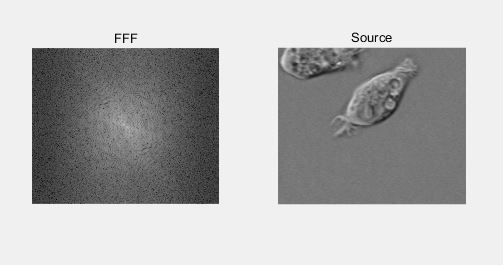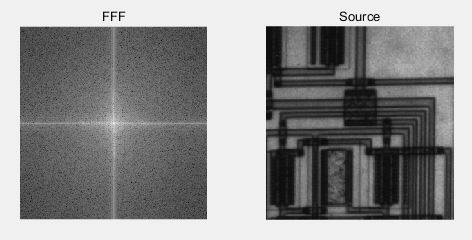美女与猫——交换两幅图像的相位谱
%求傅里叶变换 Af=fft2(A); Bf=fft2(B);
%分别幅度谱和相位谱 AfA=abs(Af); AfB=angle(Af);
BfA=abs(Bf); BfB=angle(Bf);
%交换相位谱并重建复数矩阵 AfR=AfA .*cos(BfB)+AfA .*sin(BfB) .*i; BfR=BfA .*cos(AfB)+BfA .*sin(AfB) .*i;
%傅里叶反变换 AR=abs(ifft2(AfR)); BR=abs(ifft2(BfR));
%显示图像 subplot(2,2,1); imshow(A); title('美女原图像');
subplot(2,2,2); imshow(B); title('猫的原图像');
subplot(2,2,3); imshow(AR,[]); title('美女的幅度谱与猫的相位谱组合');
subplot(2,2,4); imshow(BR,[]); title('猫的幅度谱与美女的相位谱组合');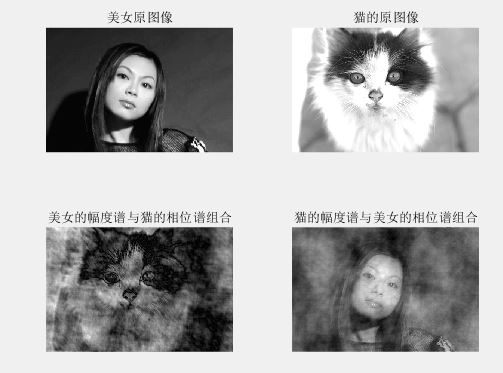4频域滤波基础
频域滤波与空间域滤波的关系：傅里叶变换可以将图像从空域变换到频域，而傅里叶反变换则可以将图像的频谱逆变换为空域图像，也即人可以直接识别的图像。
注意：将频谱原点移至图像中心，因此需要构造对应的原点在中心的滤波器，并在滤波之后使用iffshift（）函数将原点移回以进行反变换

5频率域低通滤波器
理想低通滤波器：最容易想到的衰减高频成分的方法就是在一个称为“截止频率”的位置“截断”所有的高频成分，将图像频谱中所有高于这一截止频率的频谱成分设为0，低于截止频率的成分保持不变。
高斯低通滤波器：
%生成滤镜 ff=imgaussflpf(I,20); %应用滤镜 out=imfreqfilt(I,ff);
figure(1); subplot(2,2,1); imshow(I); title('Source');
%计算FFT并显示 temp=fft2(I); temp=fftshift(temp); temp=log(1+abs(temp)); figure(2); subplot(2,2,1); imshow(temp,[]); title('Source');
figure(1); subplot(2,2,2); imshow(out); title('Gauss LPF,sigma=20');
%计算FFT并显示 temp=fft2(out); temp=fftshift(temp); temp=log(1+abs(temp)); figure(2); subplot(2,2,2); imshow(temp,[]); title('Gauss LPF,sigma=20');
%生成滤镜 ff=imgaussflpf(I,40); %应用滤镜 out=imfreqfilt(I,ff);
figure(1); subplot(2,2,3); imshow(out); title('Gauss LPF,sigma=40');
%计算FFT并显示 temp=fft2(out); temp=fftshift(temp); temp=log(1+abs(temp)); figure(2); subplot(2,2,3); imshow(temp,[]); title('Gauss LPF,sigma=40');
%生成滤镜 ff=imgaussflpf(I,60); %应用滤镜 out=imfreqfilt(I,ff);
figure(1); subplot(2,2,4); imshow(out); title('Gauss LPF,sigma=60');
%计算并显示 temp=fft2(out); temp=fftshift(temp); temp=log(1+abs(temp)); figure(2); subplot(2,2,4); imshow(temp,[]); title('Gauss LPF,sigma=60');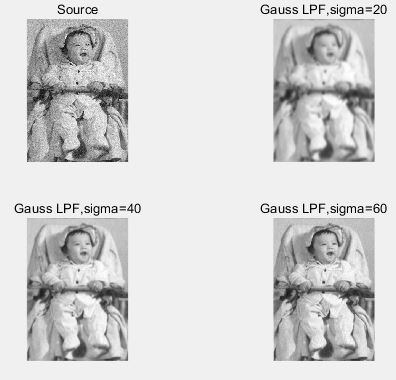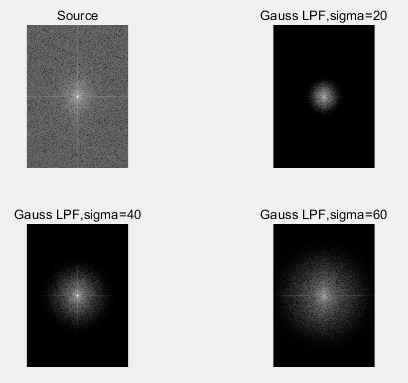6频率域高通滤波器：图像锐化可以通过衰减图像频谱中的低频成分来实现，这就建立了空间域图像锐化与频域高通滤波器之间的对应关系。
matlab实现
%生成滤镜 ff=imgaussfhpf(I,20); %应用滤镜 out=imfreqfilt(I,ff);
figure(1); subplot(2,2,1); imshow(I); title('Source');
%计算FFT并显示 temp=fft2(I); temp=fftshift(temp); temp=log(1+abs(temp)); figure(2); subplot(2,2,1); imshow(temp,[]); title('Source');
figure(1); subplot(2,2,2); imshow(out); title('Gauss HPF,sigma=20');
%计算FFT并显示 temp=fft2(I); temp=fftshift(temp); temp=log(1+abs(temp)); figure(2); subplot(2,2,2); imshow(temp,[]); title('Gauss HPF,sigma=20');
%生成滤镜 ff=imgaussfhpf(I,40); %应用滤镜 out=imfreqfilt(I,ff);
figure(1); subplot(2,2,3); imshow(out); title('Gauss HPF,sigma=40');
%计算FFT并显示 temp=fft2(I); temp=fftshift(temp); temp=log(1+abs(temp)); figure(2); subplot(2,2,3); imshow(temp,[]); title('Gauss HPF,sigma=40');
%生成滤镜 ff=imgaussfhpf(I,60); %应用滤镜 out=imfreqfilt(I,ff);
figure(1); subplot(2,2,4); imshow(out); title('Gauss HPF,sigma=60');
%计算FFT并显示 temp=fft2(I); temp=fftshift(temp); temp=log(1+abs(temp)); figure(2); subplot(2,2,4); imshow(temp,[]); title('Gauss HPF,sigma=60');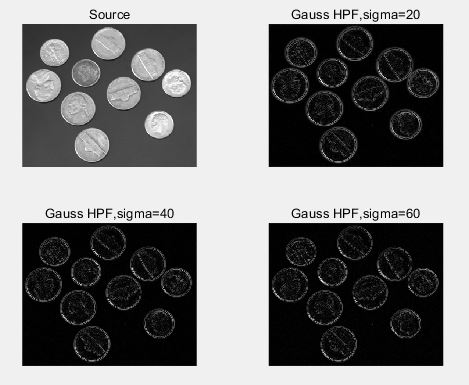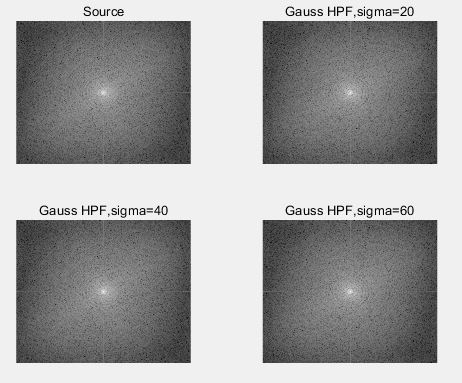高斯高通滤波器可以较好的提取图像中的边缘信息，sigma取值越小，边缘提取越不精确，会包含越多的非边缘信息；sigma取值越大，边缘提取越精确，但可能包含不完整的边缘信息。
频域拉普拉斯滤波器

ff=imlapf(I); out=imfreqfilt(I,ff);
figure(1); subplot(1,2,1); imshow(I); title('Source');
temp=fft2(I); temp=fftshift(temp); temp=log(1+abs(temp)); figure(2); subplot(1,2,1); imshow(temp,[]); title('Source');
figure(1); subplot(1,2,2); imshow(out); title('Laplace Filter');
temp=fft2(out); temp=fftshift(temp) temp=log(1+abs(temp)); figure(2); subplot(1,2,2); imshow(temp,[]); title('Laplace Filter');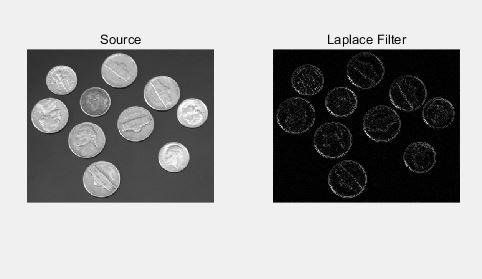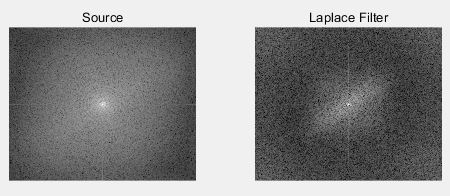7利用频域滤波消除周期噪声
频域带阻滤波器：阻止频谱中某一频带范围的分量通过，其他频率成分则不受影响。
添加周期性噪声前后对比图：
O=imread('pout.tif'); [M,N]=size(O); I=O; for i=1:M; for j=1:N;     I(i,j)=I(i,j)+20*sin(20*i)+20*sin(20*j);%添加周期噪声 end end
subplot(1,2,1); imshow(O); title('Source');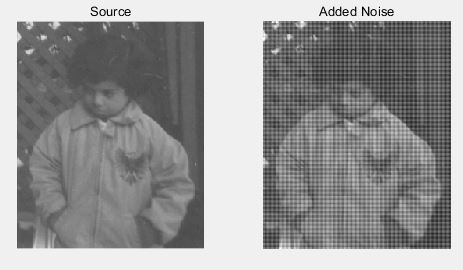频谱分析：
i_f=fft2(I); i_f=fftshift(i_f); i_f=abs(i_f); i_f=log(1+i_f);
o_f=fft2(O); o_f=fftshift(o_f); o_f=abs(o_f); o_f=log(1+o_f);
figure(1); imshow(o_f,[]); title('Source');8频率域滤波与空间域滤波之间的内在联系
频域滤波较空域滤波而言更为直观，频域下滤波器表达了一系列空域（平滑、锐化等）的本质，即对高于/低于某一特定频率的灰度变化信息予以滤除，而对其他的灰度变化信息基本保持不变。这种直观性增加了频域滤波器设计的合理性。

补充:高频与低频的区别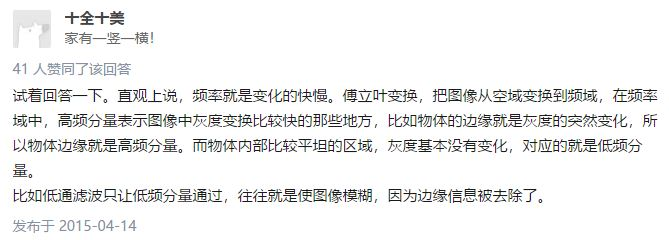展开全文• 遥感图像频率域增强,详细介绍几种图像频率域增强的方法
• 文章目录频率域图像增强1. 傅里叶变换1.1 一维傅里叶变换总结 频率域图像增强 1. 傅里叶变换 1.1 一维傅里叶变换 单变量连续函数f(x)的傅里叶变换F(u)定义为等式： 反变换可以定义为： 上述这两个式子，就反映了...


文章目录
频率域图像增强1. 傅里叶变换1.1 一维傅里叶变换1.2 二维傅里叶变换
2. 频率域滤波2.1 陷波滤波器及其性质2.2 空间域滤波与频率域滤波之间的对应关系2.2.1 空间域滤波器与频率域滤波器的转换
2.3 平滑的频率域滤波器2.3.1 理想低通滤波器2.3.2 布特沃斯低通滤波器2.3.3 高斯低通滤波器
2.4 频率域锐化滤波器2.5 频率域的拉普拉斯算子
总结

频率域图像增强
1. 傅里叶变换
1.1 一维傅里叶变换
单变量连续函数f(x)的傅里叶变换F(u)定义为等式：反变换可以定义为：上述这两个式子，就反映了通过一个函数可以做变换求到其傅里叶变换，或已知傅里叶变换可以完全地求出原始函数。
单变量离散函数f(x)（其中x=0,1,2,…,m-1）的傅里叶变换由以下等式给出：同样，若给出F(u)，能用反DFT来获得：F(u)的值的范围覆盖的域（u的值）称为频率域，因为u决定了变换的频率成分。F(u)的M项中的每一个被称为变换的频率分量。
1.2 二维傅里叶变换
二维连续函数f(x, y)的傅里叶变换F(u, v)定义为：由此可以得它的反变换：对于图像尺寸为M*N的函数f(x, y)的二维离散傅里叶变换为：给出F(u, v)，可通过反DFT得到f(x, y）如下：注：u和v是频率变量，x和y是空间或图像变量。
2. 频率域滤波
傅里叶变换的结果，与图像中的强度变化模式具有一定的联系。 例如，变化最慢的频率成分(u=v=0)对应一幅图像的平均灰度级反映的是对图像每个像素点的灰度级求和然后除以MN，得到平均灰度级。低频对应着图像的总体灰度级的显示，高频对应图像中变化快的分量（图像的细节）。
频率域滤波的步骤：
用(-1)^(x+y)乘以输入图像来进行中心变换由(1)计算图像的DFT，得到F(u，v)用滤波器函数H(u, v)乘以F(u, v)计算(3)中结果的反DFT得到(4)中结果的实部用(-1)^(x+y)乘以(5)中的结果
注：在(3)中并不是矩阵和矩阵的相乘，而是点和点的相乘。最后一步中的(-1)^(x+y)用以抵消(1)中的操作。
在MATLAB中，DFT的基本步骤如下，其中f是将被滤波的图像，g为结果，假设滤波器函数H与填充后的图像大小相同：
使用函数tofloat把输入图像转换为浮点图像：[f, revertclass] = tofloat(f);使用函数paddedsize获得填充参数：PQ = paddedsize(size(f));得到有填充图像的傅里叶变换：F = fft2(f, PQ(1), PQ(2));生成一个大小为PQ(1)*PQ(2)的滤波器函数并且令H = ifftshift(H);用滤波器乘以该变换：G = H .* F;获得G的IFFT：g = ifft2(G);将左上部的矩形修剪为原始大小：g = g(1 : size(f, 1), 1 : size(f, 2));需要时，将滤波后的图像转换为输入图像的类：g = revertclass(g);
2.1 陷波滤波器及其性质
图像的平均值由F(0, 0)给出，若在频率域中设置此项为0，并进行反变换，那么结果图像的平均值将为0。滤波函数可以选为：此滤波器可以设置F(0, 0)为零，而保留其他傅里叶变换的频率成分不变。处理后的图像可以通过对H(u, v)F(u, v)进行傅里叶反变换来获得。这一类滤波器除了原点处有凹陷外其他均为常量。
2.2 空间域滤波与频率域滤波之间的对应关系
空间域和频率域之间最基本的联系是由卷积定理的有关结论建立的。
在空间域中将滤波的模板在图像中逐像素移动，并对每个像素进行指定数量的计算的过程就是卷积过程。形式上，大小为M*N的两个函数f(x, y)和h(x, y)的离散卷积表示和定义如下：由该式可以看出，除了前面的常数、负号以及求和的上下限之外，整个表达式与空间域的线性滤波相似。特别是负号只说明函数h关于原点镜像对称。这是卷积定义中自带的。
上式是一种实现：
关于原点翻转函数通过改变(x,y)的值相对于一个函数移动到另外一个函数对每一个(x,y)的位移值，计算所有m和n值成绩的和。（(x,y)的位移值是以整数增加的，当函数不再重叠时停止）
用F(u,v)和H(u,v)分别表示f(x,y)和h(x,y)的傅里叶变换，卷积定理说明f(x,y)*h(x,y)和F(u,v)H(u,v)组成傅里叶变换对。类似的结果是频率域的卷积简化为空间域的乘法，形式上表示如下：注意，前述的所有函数均为相同尺寸。因此，在实际中，指定一个频率域的滤波器，然后进行反变换以计算相同尺寸的空间域的相应滤波器，但这种方法从计算的角度来看并不能解决太大问题。如果两个滤波器是相同尺寸，那么通常在频率域进行滤波计算更为有效。 但是，在空间域更适用于更小的滤波器。这正是我们所感兴趣的联系。滤波器在频率域中更为直观，但在空间域使用更小的滤波器模板更为明智。由此，可以在频率域指定滤波器，做反变换，然后在空间域使用结果滤波器作为在空间域构建更小的空间滤波模板的指导。
2.2.1 空间域滤波器与频率域滤波器的转换
图像处理工具箱函数freqz2可以完成空间域滤波器和频率域滤波器的等同，并输出为相应的频率域滤波器。
函数freqz2的语法形式为：H = freqz2(h, R, C)。其中，h是一个二维空间滤波器，H是相应的二维频率域滤波器。R是行数，C是我们希望滤波器H所具有的的列数。若freqz2被写成没有输出参量的形式，则H的绝对值在MATLAB桌面上显示为三维透视图。
考虑使用600*600像素的图像f，生成频率域滤波器H。
>> f = imread("C:\Users\86158\Desktop\DIP3E_Original_Images_CH04\Fig0438(a)(bld_600by600).tif");
>> imshow(f);
>> f = tofloat(f);
>> F = fft2(f);
>> S = fftshift(log(1 + abs(F)));
>> figure, imshow(S, [ ]);

如图为f和f的傅里叶频谱：图1 一幅灰度图及其傅里叶谱图2 空间域滤波和频率域滤波的比较

在空间域中使用imfilter函数生成滤波后的图像，如图2-1。在使用函数dftfilt得到频率域处理得到的滤波后的图像，如图2-2。图像中的灰色调是由于gs和gf的负数值引起的，通过标定命令imshow，负数值会使得图像的平均值增大。图2-3和2-4是将生成的sobel模板h通过使用响应的绝对值来检测图像的边缘，这样显示计算出的图像的绝对值会更有意义。在通过创建一幅经阈值处理的二值图像，可使边缘更清晰。选用乘数0.2的目的是仅显示强度比gs和gf的最大值打20%的边缘。

2.3 平滑的频率域滤波器
在空间域讨论过平滑的滤波器，需要抑制图像中的细节。而在频率域中，这些细节，如边缘和其他尖锐变化在图像灰度级中主要出于傅里叶变换的高频成分，因此频率域的平滑，可以通过衰减指定图像傅里叶变化中高频成分的范围来实现。 目标是选择一个滤波器变换函数H(u,v)以通过衰减F(u,v)的高频成分产生G(u,v)。
2.3.1 理想低通滤波器
理想低通滤波器是最简单的低通滤波器，它“截断”傅里叶变换中的所有高频成分，这些成分处在距变换原点的距离比指定距离D0要远的多的位置。其变换函数为：其中，D0是指定的非负数值，D(u,v)是(u,v)点距频率矩形原点的距离。由于变换被中心化了，如果要研究的图像尺寸为M*N，从点(u,v)到傅里叶变换中心的距离为：理想低通滤波器的模糊和振铃特性可参考卷积定理来解释。卷积定理指出在空间域的相应过程：g(x,y)=h(x,y)*f(x,y)，其中h(x,y)是滤波器变换函数H(x,y)的反变换。滤波器h(x,y)有两个主要特性：在原点处的一个主要成分，及中心成分周围呈周期性的成分。中心成分主要决定模糊，周期性的成分主要决定了理想滤波器振铃现象的特性。 中心成分的半径和距原点每单位距离上周期的数量都与理想滤波器的截止频率成反比。
下面对一幅500*500像素的图像f应用一个理想低通滤波器处理结果如下：
>> Fimg=fft2(double(f));
>> Fimg=fftshift(Fimg);
>> [M,N]=size(f);
>> dist1=5;
>> z1=zeros(M,N);
>> for i=1:M
for j=i:N
if(sqrt(((i-M/2)^2+(j-N/2)^2))<dist1)
z1(i,j)=1;
end
end
end
>> g1=Fimg.*z1;
>> g1=ifftshift(g1);
>> img1=real(ifft2(g1));
>> imshow(img1);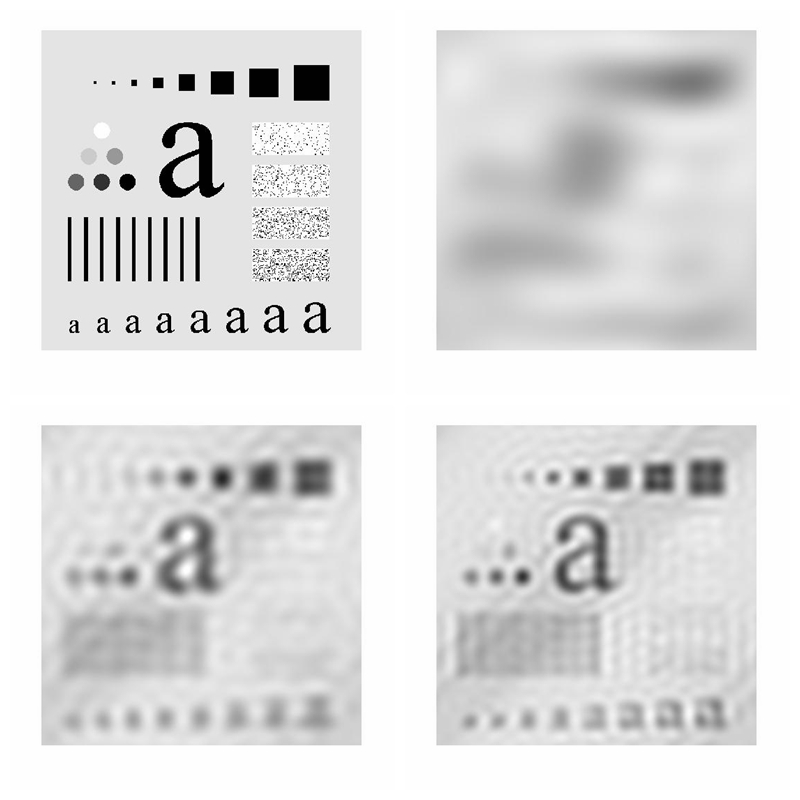图3 理想低通滤波器处理后的图像

由图可以看出，图3-2对所有的实际目的没有意义，除非模糊的目的是为了消除所有的细节。随着滤波器半径的增大，消除的功率越来越少，导致的模糊也越来越弱。可以明显看出，在图3-3和3-4中，有振铃的效果存在，随着被消除的高频部分的数量减少，图像的纹理变得越来越清晰，在半径更大的处理中，滤波的截止频率很大，虽然有振铃存在，但是没有那么明显了。
2.3.2 布特沃斯低通滤波器
n阶布特沃斯低通滤波器(BLPF)的传递函数（且截止频率距原点的距离为D0）的定义如下：布特沃斯低通滤波器变换函数在通带与被滤除的频率之间没有明显的截断。 一个一阶布特沃斯滤波器没有振铃，在二阶中振铃通常很微小，但阶数增高时振铃便成为一个重要因素。相比于理想低通滤波器，布特沃斯滤波器减少了振铃现象，高低频率之间的过渡比较平滑。但其平滑处理的效果常不如理想低通滤波器。需要根据平滑效果和振铃现象这种选择布特沃斯滤波器的阶数。并且其计算量大于理想低通滤波器。
2.3.3 高斯低通滤波器
二维高斯低通滤波器(GLPF)的形式由下式给出：其中，D0是截止频率。当D(u,v)=D0时，滤波器下降到它最大值的0.607处。高斯滤波器有一个重要的特性，那就是在高斯滤波器中是没有振铃的。其平滑效果常不如布特沃斯低通滤波器。
下面对一幅500*500像素的图像f应用一个高斯低通滤波器，D0的取值分别为5,15,30和230，处理结果如下：
>> [f, revertclass] = tofloat(f);
>> [U, V] = dftuv(PQ(1), PQ(2));
>> D = hypot(U, V);
>> D0 = 0.05 * PQ(2);
>> F = fft2(f, PQ(1), PQ(2));
>> H = exp(-(D.^2)/(2 * (D0^2)));
>> g = dftfilt(f, H);
>> g = revertclass(g);
>> imshow(g);图5 高斯低通滤波处理后的图像

对比原来的图像，相比于理想低通滤波和布特沃斯滤波，没有其模糊的严重，并且没有振铃的情况存在。一个图像在频率域是高斯函数，在空间域同样也是高斯函数。
2.4 频率域锐化滤波器
灰度级的边缘和其他地方的急剧变化与高频成分有关，图像的锐化能够在频率域用高通滤波处理实现，而衰减低频成分并不会扰乱傅里叶变换的高频信息。
高通滤波器，可以通过对低通滤波器进行精确的反操作来得到。
2.5 频率域的拉普拉斯算子
频率域的拉普拉斯算子可由如下滤波器实现：但这个公式在处理数字图像时，还需进行比例的变换。例如对于大小为M*N的数字图像f(x, y)，实际的频率域拉普拉斯算子为：如果不做这个处理，u和v的取值范围从0至M-1和0至N-1的，u^2 + v^2其实是一个非常大的值，这样不适合在数字图像中做处理的。
计算F(u,v)时需要先中心化，也就是将中心移到M/2和N/2处，则此时为：总结
在这一部分，仅处理有限域内的函数（图像），傅里叶技术提供了一个有意义的和实际的研究以及实现图像增强的主要途径。
为什么要在频率域研究图像增强？可以利用频率成分和图像外表之间的对应关系。一些在空间域表述困难的增强任务，在频率域中变得非常普通；滤波在频率域更为直观，它可以解释空间域滤波的某些性质；可以在频率域指定滤波器，做反变换，然后在空间域使用结果滤波器作为空间域滤波器的指导；一旦通过频率域滤波试验选择了空间滤波，通常实施都在空间域进行。
频率域滤波是基于傅里叶变换所做的处理，它对傅里叶变换后产生的反映频率信息的图像进行处理，主要有频率域低通滤波器和频率域高通滤波器。
由于图像中的噪声主要集中在图像的高频部分，为了去除噪声，改善图像质量，可以采用低通滤波器来抑制高频部分，然后再进行傅里叶反变换获得滤波图像，可以达到图像平滑的目的，常用的频率域低通滤波器有理想低通滤波器、布特沃斯低通滤波器和高斯低通滤波器。这三种滤波器中理想滤波器平滑效果最好，但是高斯滤波器中没有振铃现象。通过对低通滤波器的反操作，可以得到高通滤波器。
展开全文计算机视觉...